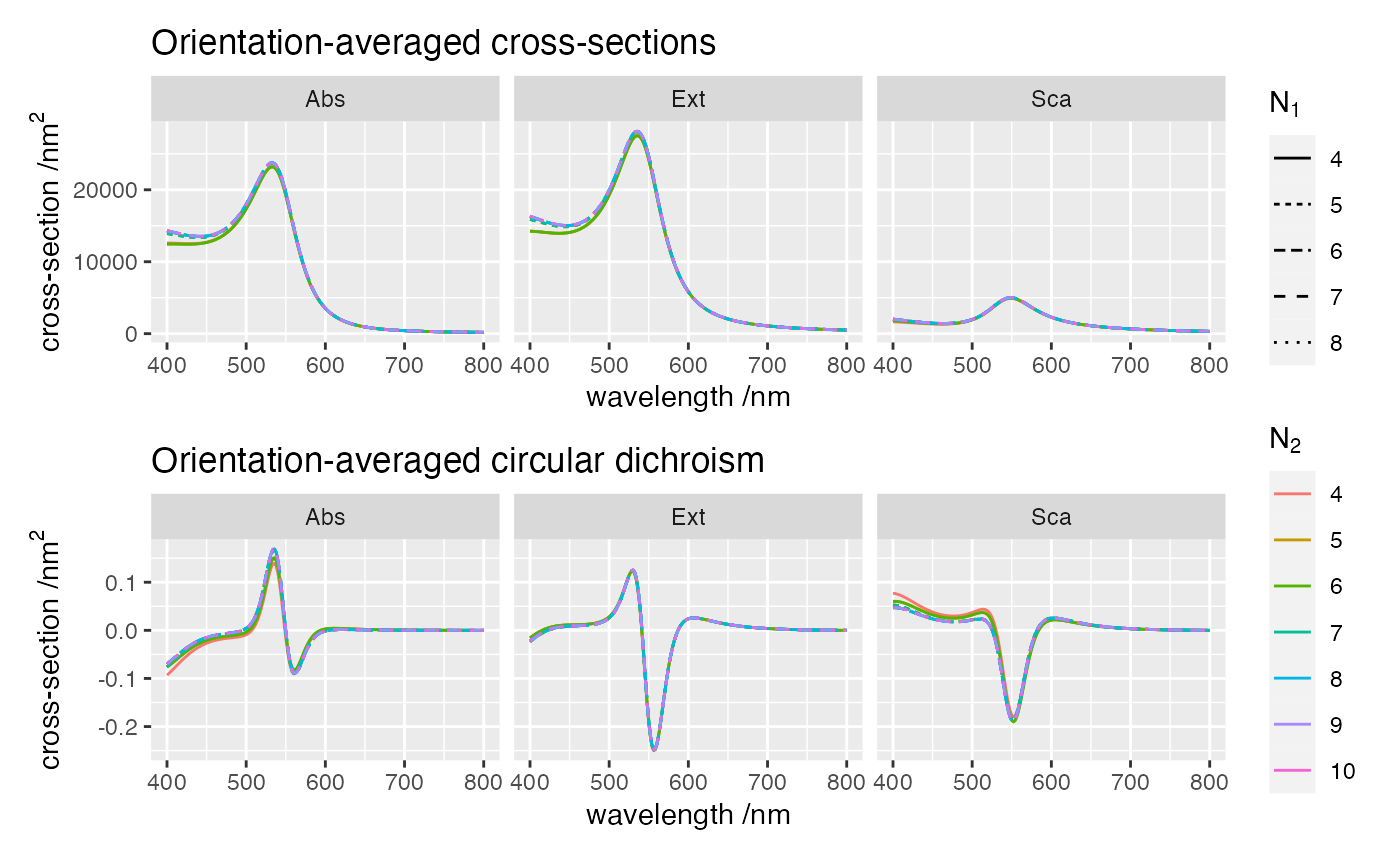## Objective

This example checks the convergence of orientation-averaged cross-sections with respect the maximum multipolar order describing individual scatterers and translation of vector spherical waves. The structure consists of four gold spheres in water arranged in a helix.

This simulation uses the following input file

ModeAndScheme 2 3
Wavelength 400 800 200
Medium 1.7689 # epsilon of water
MultipoleSelections 1
EE1:4_EM1:4_ME1:4_MM1:4 blocks
OutputFormat HDF5 cross_sections_{n1}_{n2}

MultipoleCutoff {n1} {n2}

Scatterers 4
Au_S1 200 0 -75 25
Au_S1 161.803398874989 117.557050458495 -25 25
Au_S1 61.8033988749895 190.211303259031 25 25
Au_S1 -61.8033988749895 190.211303259031 75 25

where {n1}, {n2} are replaced by 4,5,... in each separate run.

## Orientation-averaged results

We see that in this example the cross-sections require higher than 4 multipolar orders in both $$n_1$$ and $$n_2$$ to converge, especially at shorter wavelengths. Typically, one needn’t set $$n_2$$, as the default value $$n_1=n_2$$ is accurate; this optional parameter is mostly a remnant from the initial development stages.Last run: 23 February, 2022